# Fractions & Decimals Worksheets for Grade 3

## Free fraction and decimals worksheets

Our grade 3 fractions and decimals worksheets provide practice exercises on introductory fraction and decimal concepts, including identifying simple fractions, equivalent fractions and simple fraction and decimal addition and subtraction.  Several worksheets (with answer keys) are provided for each type of exercise.

## Identifying fractions

Example

Identify fractions - color in the fraction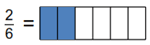Identify fractions - write the fraction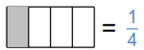Fractional part of a set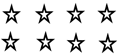## Equivalent fractions

Equivalent fractions - color in the fraction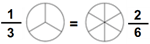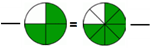Equivalent fractions (numerators missing)

__ /3 = 20/30

Equivalent fractions (harder version)

6/8 = __ /56

Equivalent fractions (numerators or denominators missing) 2/3 = 10/__
3 equivalent fractions 1/3 = 3/__  = 6/__

## Comparing fractions

Compare 2 fractions, same denominator (with pie charts)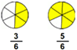Compare 2 fractions, different denominators (with pie charts)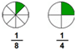Compare 2 proper or improper fractions (with pie charts)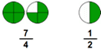Compare mixed numbers and fractions (with pie charts)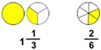Comparing fractions (like denominators) 4/5 > 3/5
Comparing fractions (unlike denominators) 9/10 > 1/2
Comparing fractions (improper fractions) 3/2 > 3/4
Comparing fractions (mixed numbers) 1 1/2 < 3 1/6

## Simplifying fractions

Simplifying fractions (proper fractions) 20/24 =
Simplifying fractions (proper and improper fractions) 26/10 =

1/6 + 2/6 =

3 1/6 + 4 2/6=
1/2 + ___ = 2
Completing whole numbers (mixed numbers) 3 1/3 + ____ = 4

3/10 - 1/10 =
Subtracting improper fractions (like denominators) 8/6 - 5/6 =
Subtracting a fraction from whole number 6 - 2/3 =
2  4/5 - 1/5 =
Subtract a mixed number from a whole number 10 - ___ = 5 3/4
Subtract mixed numbers (like denominators) 8 3/7 - 3 6/7 =

## Converting fractions to / from mixed numbers

Mixed numbers to fractions 9 1/5 =
Fractions to mixed numbers
46/5 =

## Converting fractions to / from decimals

Decimals to mixed numbers 7.3 =
Fractions to decimals 1/10 =
Mixed numbers to decimals 1 1/10 =

## Comparing and ordering decimals

Comparing decimals 0.9  <  1.2
Ordering decimals 9.3, 42.7, 89, 706

1 decimal digit - easy 0.8 + 1.8 =
Add in columns (1 decimal digit)

0.8

+ 1.8

## Decimal Subtraction

Subtract 1-digit decimals from whole numbers 8 - 0.8 =
Subtract 1-digit decimals from whole numbers (missing minuend/subtrahend) 10 - ___ = 9.1

50.0

- 3.8

## Word problems

Fraction Word Problems for Grade 3